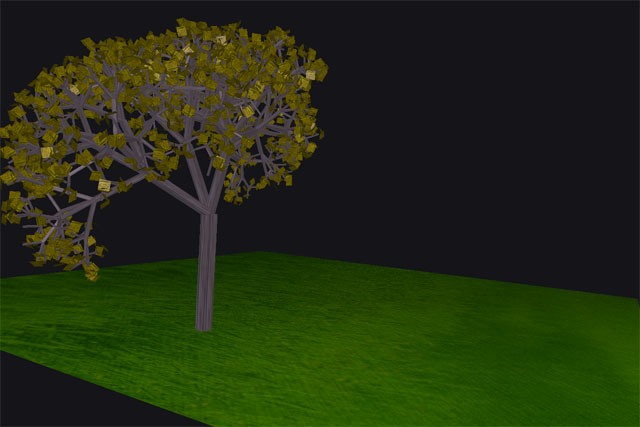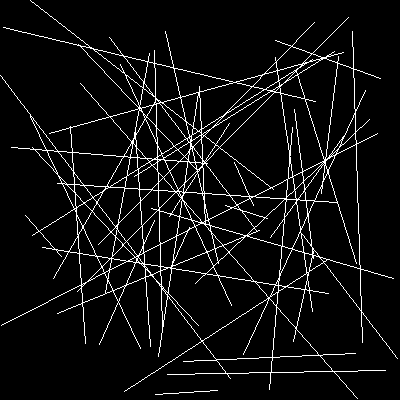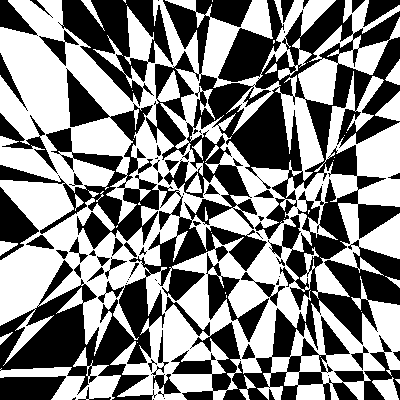Recursion (you have seen it before)• Asexual reproduction

BacteriaPlan: grow yourself, when you are large enough split in two. Each of you now follows the BacteriaPlan.

• Sexual reproduction

PeoplePlan: grow yourself, when you are old enough, repeat: find someone to have a baby with and have a baby. The baby follows the PeoplePlan.

• Ponzi scheme (as in Madoff arrested in alleged Ponzi scheme
• Pyramid scheme: The Plan: Find 2 people (p and q) Sell this plan to them for \$4 Make sure that p and q follow this plan Send 50% of what p and q send you to the person that sold you this plan.

Problem Solving with Recursion

Recursion is a problem solving technique which uses recursion to solve problems

In general it looks like... def solve(p): if p is simple enough: s=simple solution to p else: break p into problems p1,...,pk where each of p1,...,pk 'looks like' p each of p1,...,pk is 'simpler' than p s1=solve(p1) # recursive call to solve s2=solve(p2) # recursive call to solve . . . sk=solve(pk) # recursive call to solve # combine the solutions to the smaller problems # into a solution s for p (our current problem) s=combine(s1,...,sk) return s
• Consider a checkerboard which has dimensions 2^2000 by 2^2000. Now remove 1 square. You want to tile the checkerboard with L shaped tiles. You must not cover the 'removed' square. Prove that, no matter which square you remove, you can always completely tile the resulting checkerboard. A tile looks like ... o oo
• Prove that an arrangement of n lines in the plane can be colored using at most 2 colors. You are coloring the regions bounded by the lines. No edge can have the same color on both sides. That is, two adjacent regions (sharing an edge) can not have the same color.twoColour.py
• Print a triangle A of height n recursively x xx xxx xxxx xxxxx xxxxxx
• Print a triangle V of height n recursively xxxxxx xxxxx xxxx xxx xx x triangles.py
• Why are spirals and trees recursive? drawingRecursively.py• Print 1 to n recursively. Print n to 1 recursively. Sum up numbers from i to j recursively. sum.py
• McNugget problem (which amounts are buyable): In McDonalds, you can purchase packs of 4, 6 and 10 McNuggets. Call a number n buyable if you can purchase packs summing up to a total of exactly n McNuggets. Clearly 4,6 and 10 McNuggets are buyable. 16 is buyable (10+6). Is 23 buyable? Is 197 buyable? Is n buyable?
• How do I sort recursively? Searching and Sorting